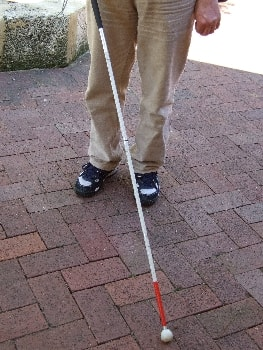## Definition

Luminance Contrast is defined as the light reflected from one surface or component, compared to the light reflected from another surface or component. To undertake an assessment one must first determine the luminous reflectance values (LRV) of each surface being considered.

The luminous reflectance values are also known as the ‘Y’ value of the CIE colour system.

## Testing Methodology

LumiLab’s methodology in measuring the LRVs is outlined in:

• Appendix B of AS 1428.1 (2009); and
• Appendix E of AS 1428.4.1.

Building elements and TGSIs tested in the LumiLab laboratories to determine the LRVs are then assessed using the Bowman-Sapolinski equation to compare the two LRVs to determine the luminance contrast.

## Comparing LRVs

In fact, anyone can determine a luminance contrast if you know the two tristimulus value Ys in the CIE colour system, these are referred to as the LRVs. Just put the Y1 and Y2 values into the Bowman-Sapolinski equation below, or use our free calculator:

C = 125 (Y2 − Y1)/(Y1 + Y2 + 25)where:

• C = luminance contrast
• Y1 and Y2 = luminous reflectance values (or LRV) of the two surfaces